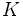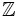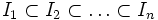# Dedekind domain

This article defines a property of integral domains, viz., a property that, given any integral domain, is either true or false for that.
View other properties of integral domains | View all properties of commutative unital rings
VIEW RELATED: Commutative unital ring property implications | Commutative unital ring property non-implications |Commutative unital ring metaproperty satisfactions | Commutative unital ring metaproperty dissatisfactions | Commutative unital ring property satisfactions | Commutative unital ring property dissatisfactions

## Definition

### Symbol-free definition

An integral domain is termed a Dedekind domain if it satisfies the following equivalent conditions:

## Relation with other properties

### Stronger properties

Property Meaning Proof of implication Proof of strictness (reverse implication failure) Intermediate notions
principal ideal domain integral domain in which every ideal is a principal ideal Dedekind not implies PID click here
polynomial ring over a field ring of the form$K[x]$ where$K$ is a field click here
ring of integers in a number field it is the integral closure of$\mathbb{Z}$ inside a number field (a finite degree field extension of the rationals) click here

### Weaker properties

Property Meaning Proof of implication Proof of strictness (reverse implication failure) Intermediate notions
one-dimensional ring the Krull dimension is at most one, i.e., we cannot have a strictly ascending chain$P_0 \subset P_1 \subset P_2$ of prime ideals click here
one-dimensional domain integral domain and a one-dimensional ring. Explicitly the prime ideals are precisely the zero ideal and nonzero maximal ideals. click here
one-dimensional Noetherian domain integral domain that is a Noetherian ring and a one-dimensional ring. click here
Noetherian normal domain integral domain that is a Noetherian ring and a normal ring. click here

### Conjunction with other properties

Any unique factorization domain which is also a Dedekind domain, is also a principal ideal domain.

## Module theory

Any finitely generated module$M$ over a Dedekind domain$R$ can be expressed as a direct sum as follows:$M \cong R/I_1 \oplus R/I_2 \oplus \ldots \oplus R/I_n$

where$I_1 \subset I_2 \subset \ldots \subset I_n$ is an ascending chain of ideals, which could reach$R$.